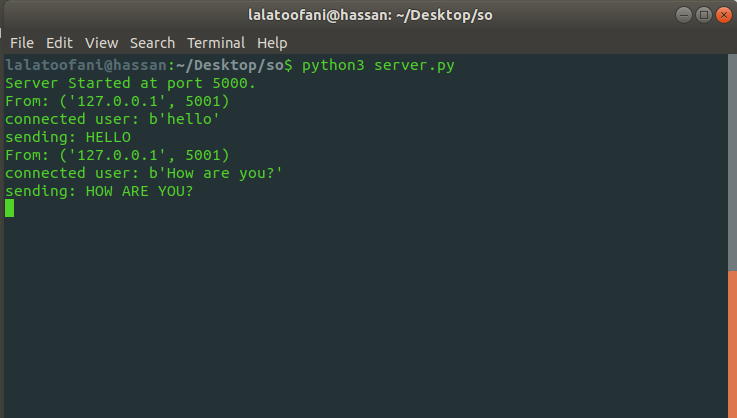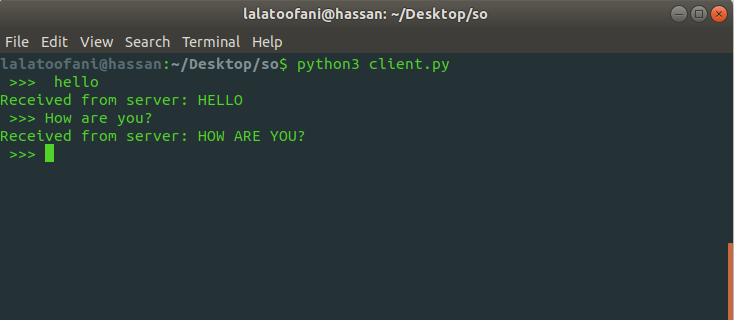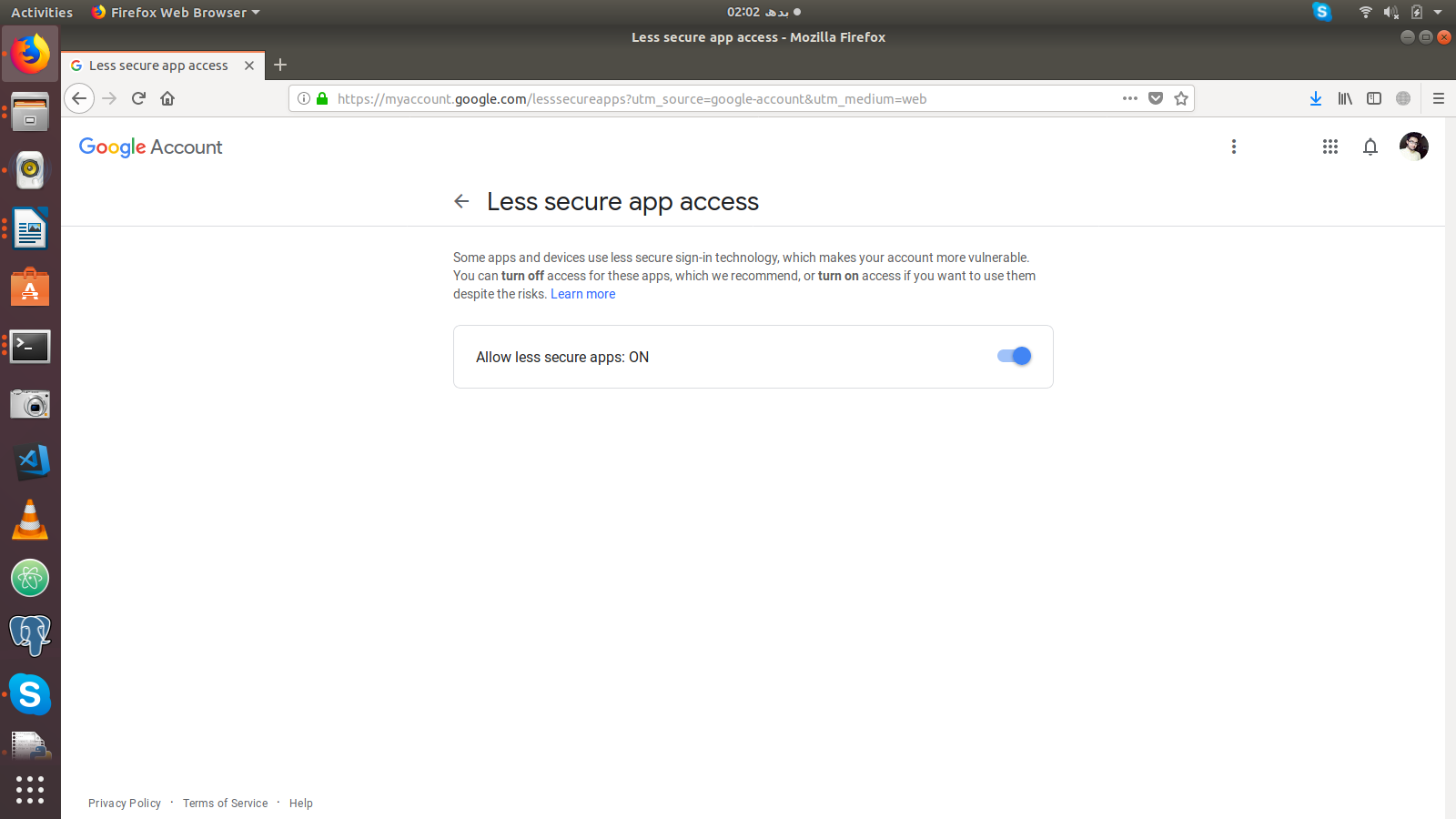## Practical Implementation of UDP and SMTP in Python

##### In this part of Learning Python we Cover Implementation of UDP and SMTP in Python
Written by |25-Apr-2019 | 0 Comments | 357 Views

In previous section we were discussing about UDP. Now we will write the code and see what is happening at each step:

`Client.py`
`import socket`
`def Client():`
`    host = '127.0.0.1'`
`    port = 5001`
`    server = ('127.0.0.1',5000)`
`    sock = socket.socket(socket.AF_INET, socket.SOCK_DGRAM)`
`    sock.bind((host, port))`
`    message = input(" >>>  ")`
`    while message != 'q':`
`        sock.sendto(message.encode('utf-8'), server)`
`        data, addr = s.recvfrom(1024)`
`        print ('Received from server: ' + str(data.decode('utf-8')))`
`        message = input(" >>> ")`
`    sock.close()`

`if __name__ == '__main__':`
`    Client()`

The above mention code is of client py. In this file, we first import the socket module as usual.

• In the next step, we make a function named client and assign the host address and port number.

• We have assigned server variable which is the exact address of the server the above host and port is the new port to send the data. If you are using it on 2 system separately than you can use the same port of 5000 otherwise, it will give an error of port already in use,

• In the third step we initialize the socket. Here is the difference between TCP and UDP initialization. Here we have given two parameters. We will discuss both of them:

AF_INET:

AF_INET is an address family that specifies the type of address a socket going to use. Here we are using internet protocols like IPv4 or IPv6.

SOCK_DGRAM:

SOCK_DGRAM is an attribute which is given in class initialization of socket to specify that following program, is going to use UDP protocol.

• After initialization, we bind the address by providing it with host and port in a tuple.

• It is to be noted that there is no accept function we use in UDP because UDP is a connectionless server protocol which doesn’t use acknowledgments.

• Now the user writes the message if the message does not equal to q then the message will be sent to the server. The data can also be received at the same time.

`Server.py`
`import socket`
`def Server():`
`    host = '127.0.0.1'`
`    port = 5000`
`    s = socket.socket(socket.AF_INET, socket.SOCK_DGRAM)`
`    s.bind((host,port))`
`    print (f"Server Started at port {port}.")`
`    while True:`
`        data, addr = s.recvfrom(1024)`
`        print ("From: " + str(addr))`
`        print ("connected user: " + str(data))`
`        data = str(data.decode('utf-8')).upper()`
`        print ("sending: " + str(data))`
`        s.sendto(data.encode('utf-8'), addr)`
`    c.close()`

`if __name__ == '__main__':`
`    Server()`

• All the steps are the same except the code in the while block. In while block the data is received from the client and program changes into upper case and send back to the client which ensures that server is running successfully.

•  Following figures below shows the program running locally:Figure 1Figure 2

The above figures are of server and client running on two different terminals. When a client sends any message, it converted it into upper case and sent back to the client if you want to connect on 2 different laptops or PC for that you have to give proper IP address which can be known by the writing ifconfig in a terminal.

SMTP:

SMTP is another internet protocol used to send emails. It stands for Simple Mail Transfer Protocol. In python, there is a module available that make it possible to send the email via script. This makes it easy for online verification and mailing system for a company like a mobile phone which sends multiple emails to users at the same time; otherwise, it will be hard to handle such a big audience.  To use SMTP python, you must have the GMAIL mailing account. If you don’t have one then create one, it’s straightforward to create an account.

After you are logged in than go to url mentioned belowFigure 3

and on the less secure app as show in Figure 3. Also install smtplib via pip command.

After all these steps we are good to go to send an email through python script.

Sending Plain Text Message:

In the first example we will see how to send the plain text message via SMTP in python. Using following method:

`Code`

`from email.mime.multipart import MIMEMultipart`
`from email.mime.text import MIMEText`
`import smtplib`
`Msg_Obj = MIMEMultipart()`
` `
`message = "Hey whats up doc!!!"`
`password = "**************"`
`Msg_Obj['From'] = "sender@gmail.com"`
`Msg_Obj['To'] = "receiver@anything.com"`
`Msg_Obj['Subject'] = "Testing my first SMTP python script"`
`Msg_Obj.attach(MIMEText(message, 'plain'))`
`server = smtplib.SMTP('smtp.gmail.com: 587')`
` `
`server.starttls()`
`server.login(Msg_Obj['From'], password)`
`server.sendmail(Msg_Obj['From'], Msg_Obj['To'], Msg_Obj.as_string())`
`server.quit()`
` `
`print (f"Email sent at {Msg_Obj['To']}")`

The above code is of sending the clear message. We first initiate the object of and then we have to give the credentials and receiver information and the message that we want to send. After all this, we have to initialize the SMTP server. It is to be noted that SMTP server works only on 587 port if you change the port than the server will not work.

After initializing the server, we will log in to the server by the credentials declared in the above variables and then the server will send message to the mailing address.

# Send Image via SMTP:

As we see in the above code, we have sent the plain text similarly we can send the image via SMTP. Following is the code of sending the image via SMTP:

`Code`

`from email.mime.multipart import MIMEMultipart`
`from email.mime.text import MIMEText`
`from email.mime.image import MIMEImage`
`import smtplib`
`Msg_Obj = MIMEMultipart()`
`with open('pylogo.png', 'rb') as fp:`
`    img = MIMEImage(fp.read())`
`message = "Image is attach in a mail"`
`password = "shrpwd11993730"`
`Msg_Obj['From'] = "the.adonis119@gmail.com"`
`Msg_Obj['To'] = "shr119@outlook.com"`
`Msg_Obj['Subject'] = "Testing Image via SMTP"`
`Msg_Obj.attach(MIMEText(message, 'plain'))`
`Msg_Obj.attach(img)`
`server = smtplib.SMTP('smtp.gmail.com: 587')`
` `
`server.starttls()`
`server.login(Msg_Obj['From'], password)`
`server.sendmail(Msg_Obj['From'], Msg_Obj['To'], Msg_Obj.as_string())`
`server.quit()`
` `
`print (f"Email sent at {Msg_Obj['To']}")`

he above code can import additional module which is MIMEImage which is used to attach the image with the message object. Moreover, then this message will be sent via SMTP.

Similarly, we can send html by adding the html as payload and assigning the header as follows:

In that way, we can send the HTML too by giving the HTML in the payload. We can assign and design as we want to.